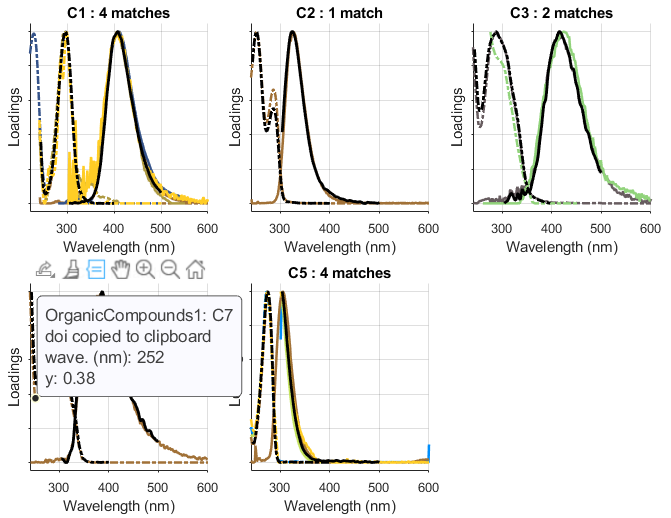PARAFAC tutorial (pure fluorophores)

This is a tutorial that will demonstrate how to

• fit PARAFAC models to an EEM dataset
• identify and handle outlier samples and deviating EEM spectra
• validate models
• export the PARAFAC results

This tutorial uses a dataset consisting of pure fluorophores that were mixed in silico. The EEMs depicted here therefore truly behave according to Beer-Lambert, and Kasha’s rule, and do not violate any of the assumptions of the PARAFAC model. This tutorial therefore focuses on understanding how to fit, validate, and export PARAFAC models. It does not address other challenges that are typically encountered when fitting models to unknown compound mixtures where disturbances can occur and invalidate the application of PARAFAC (e.g. large variations in pH, ionic strength, solvent composition, spectral misalignment).

The dataset does contain outliers. These are samples that contain either emission or excitation scans that “went wrong”, i.e. are either disproportionally high or low.

You can copy & paste the code sections in this tutorial directly into your own MATLAB script. If this document is open in the MATLAB help browser (called with `doc`), you can right-click on code sections and evaluate them line-by-line (also by pressing `F9` on windows).

If you want the code without any comments given in this tutorial, type:

``````open(which('drEEM_parafac_tutorial_wuensch.m'))
``````

The drEEM toolbox must be installed for this command to work.

The sections of this tutorial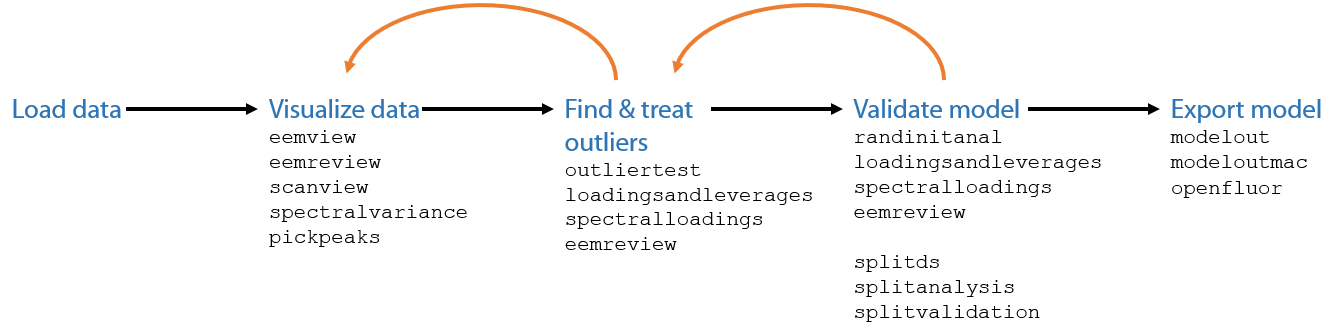Most of the tutorial focuses on outlier tests. This is intentional and I made sure to hide some challenges in this dataset. A good model describes good data. If issues exist in the raw data, we often settle for models with less components as the issues tend to have a smaller impact. Proper visualization and focus on outlier treatment can make a big difference and you will obtain much better modeling results.

## Set up the toolbox

Before we start this tutorial, we need to make sure that the drEEM toolbox is properly installed and the functions are ready to import a example dataset. This set of data is called `tutorial_dataset2.mat` and is stored in the drEEM toolbox folders.

If you downloaded the drEEM toolbox to your matlab user folder (the folder returned when you call `userpath`), simply call

``````cd userpath
``````

otherwise, say:

``````cd 'C:\Users\expertuser\somefolder\drEEM\' % Change this!
``````

then, we ensure proper installation of drEEM and availability of the demo files:

``````dreeminstall
``````

## Load the dataset and visualize the data

The first step in any PARAFAC analysis is to get to know your data. It may be tempting to simply import data and “run the PARAFAC”, but you will most likely obtain a bad model or settle for a three-component model instead of a seven-component model because “the split-validation did not work”.

First, let’s load the data and take a look at what it contains:

``````load(which('tutorial_dataset2.mat'))
disp(sprintf(DS.description{:}))
``````

We obtain this information:

``````>> disp(sprintf(DS.description{:}))
drEEM PARAFAC tutorial dataset. (c) Urban Wuensch, 2018.
This dataset consists of 60 samples in which pure fluorophores were added at random to make EEM spectra.
The dataset contains random noise typical for an Horiba AquaLog spectrofluorometer.
As this is an artifical sample dataset, a correct solution for the number of components exists.
Contact the author (urbw@aqua.dtu.dk or wuensch@chalmers.se) if you have questions or comments.
``````

To see the fields of `DS`, simply type `disp(DS)`

This dataset is correctly formatted, but you can check yourself by typing `checkdataset(DS)`. Next, we should see what our EEMs look like:

``````eemview(DS,'X',[3 3])
eemreview(DS)
scanview(DS)
spectralvariance(DS)
``````

We can see that these EEMs do not resemble DOM fluorescence. The emission drops beyond 470nm, and we see not a lot of absorbance at excitation >340nm. But there are quite a lot of fluorescence peaks.

This dataset is also fully corrected. EEMs do not contain traces of pysical scatter. If you want to learn more about how to excise scatter, please refer to our scatter removal tutorial.

## Outlier tests

From the call `spectralvariance(DS)`, we can see right away that our dataset may contain outliers. We can fit some rough PARAFAC models to confirm our suspicion and identify the outlier samples. The function `outliertest` does this for us.

`outliertest` fits two models with very low convergence criterion and various number of components to your data. Type `doc outliertest` to learn more.

Test1=outliertest(DS,3:6);

This function produces some graphical output. You can obtain these plots again by calling `outliertest(Test1,[],3:6)`. Here, we focus on the six-component model, as it is the one that is most vulnerable to suffer from outliers. Models with lower numbers of components often average spectral features. This makes them “overlook” outlier scans or samples to some degree.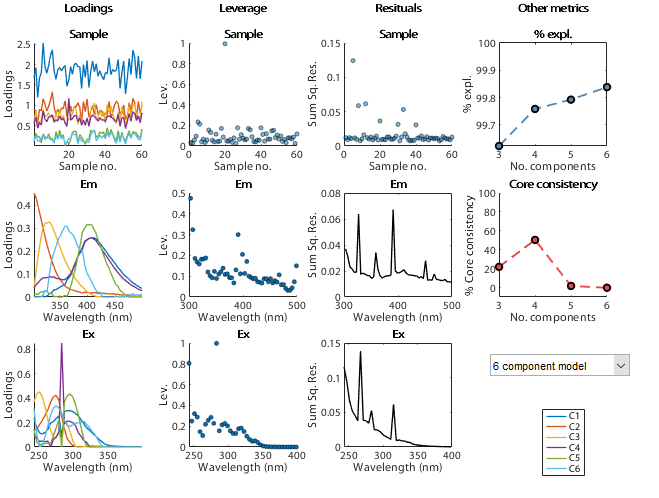We can see from the plots, that some samples have a very high leverage or high residual signals. Sample 20 stands out most. Take a look at it with `eemreview(DS,'samples',)`. It contains an excitation scans that appears abnormally high. We can use `zap`, to address this. Note down which excitation scans they are and “zap” these data. Type `doc zap` to learn more.

``````DS=zap(DS,20,[],283);
``````

Now, we repeat the outliertest.Remember: PARAFAC requires an iterative approach where we sometimes have to go back one step after correcting issues.

``````Test2=outliertest(DS,[],3:6);
``````

After taking a look at the diagnostics of the six-component model, it appears that more samples (5, and 33) have a large influence on the model outcome. Why? Answer: `eemreview(DS,'samples',[5 33])`. Solution:

``````DS=zap(DS,5,[],267);
DS=zap(DS,33,451,247);
DS=zap(DS,33,327,[]);
``````

And again:

``````Test3=outliertest(DS,[],3:6);
``````

Now, sample 8 appears to have a large leverage. Why? Answer: `eemreview(DS,'samples',)`. Solution:

``````DS=zap(DS,8,[],315);
``````

And we try again:

``````Test4=outliertest(DS,[],3:6);
``````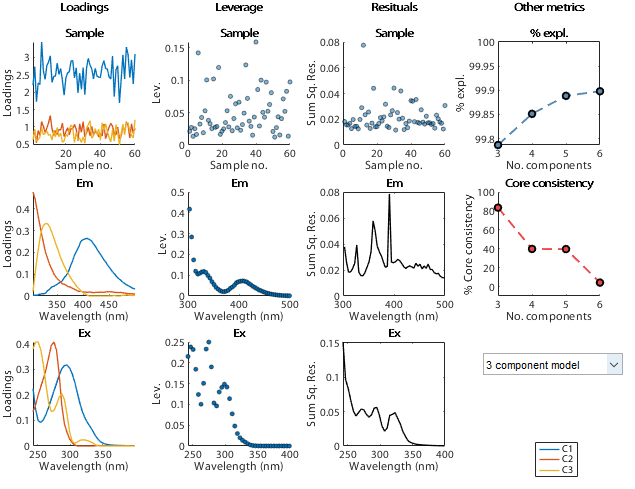After this 4th test, we see one sample with a high leverage (12), and two samples with high residuals (30 and 40). Use `eemreview` to take a look at them and zap the questionable areas.

``````eemreview(DS,'samples',[12 30 40])
DS=zap(DS,12,391,[]);
DS=zap(DS,30,359,[]);
DS=zap(DS,40,327,[]);
DS=zap(DS,40,363,315);
``````

Again, one more outliertest:

``````Test5=outliertest(DS,[],3:6);
``````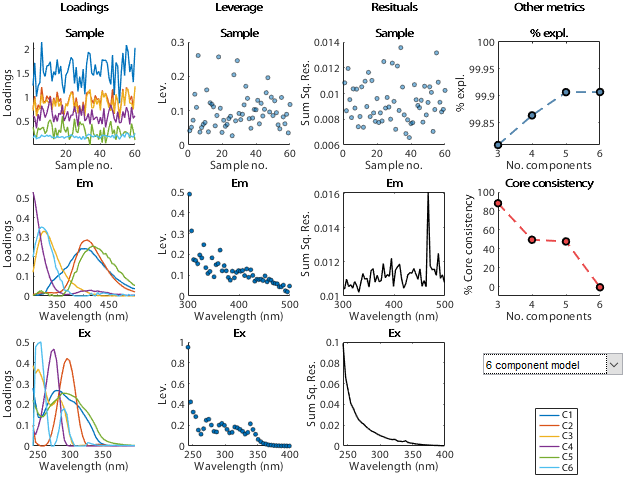The result looks good. Leverages are quite equal between samples, the leverages of exitation and emission follow spectral shapes and are quite smooth, and residuals are quite random. Some peak shapes in residuals are to be expected as this is a very preliminary model. At this stage, we are looking for any signs that samples or wavelengths are different from their neighbors.

If you run this tutorial and do not obtain the same preliminary models, remember that outliertests are rough models. The outcome can be quite different from run to run.

Note

Instead of `outliertest`, we could have:

1. Flipped through each of the 60 EEMs with `eemview(DS,'X',[3 3])`
2. Noted down sample numbers of strange EEMs
3. Used `eemreview(DS,'samples',[outlier1 outlier2...])` to note down which wavelengths are to be removed
4. Used `zap` to remove the scans like shown above.

These two strategies are both valid, and the manual one may be a bit more robust as it does not require good preliminary PARAFAC models. `outliertest`-models can sometimes indicate issues where there are non (we had an example above with sample 30. This is due to the low convergence criterion used in `outliertest`. A more robust solution can be obtained with `randinitanal`, but this also takes longer.

In your own dataset, you may not always know why a certain sample has a high leverage as it looks “normal”. If that is the case, you can use `subdataset` to exclude the entire sample, instead of zapping only some scans. Often, these samples feature fluorescence that is somehow very different from the remainder of the samples. PARAFAC finds common patterns in datasets, so a sample with different fluorescence is a true “outlier”.

Always take a look at high-leverage samples. You should know why you exclude them or zap some scans. “Because it had a high leverage” is not a good reason.

## Inspect model parameters

Now that we have checked for outliers, the next question is: How many components do I need to describe the fluorescence of these 60 EEMs? While in early versions of drEEM, this question may have been addressed with `outliertest`, drEEM supports parallel computing since v0.5.0 and we thus encourage fitting more models with lower convergence criteria to get a more reliable indication for answering the component-number question. This can be done with `randinitanal`. Type `doc randinitanal` to learn more about this function. Here, we don’t quite know that to do, so we fit two to seven components and we start each model 10 times to identify the best out of 10 solutions. As fluorescence is generally a phenomenon that is observed as positive signal, we can constrain the model to positive scores and loadings. This is termed `nonnegativity` in PARAFAC.

This next command will take some time to complete. The newer your MATLAB and the more powerful your computer, the faster it will finish.

``````models=randinitanal(DS,2:7,10,'nonnegativity');
``````

You will see an output like this: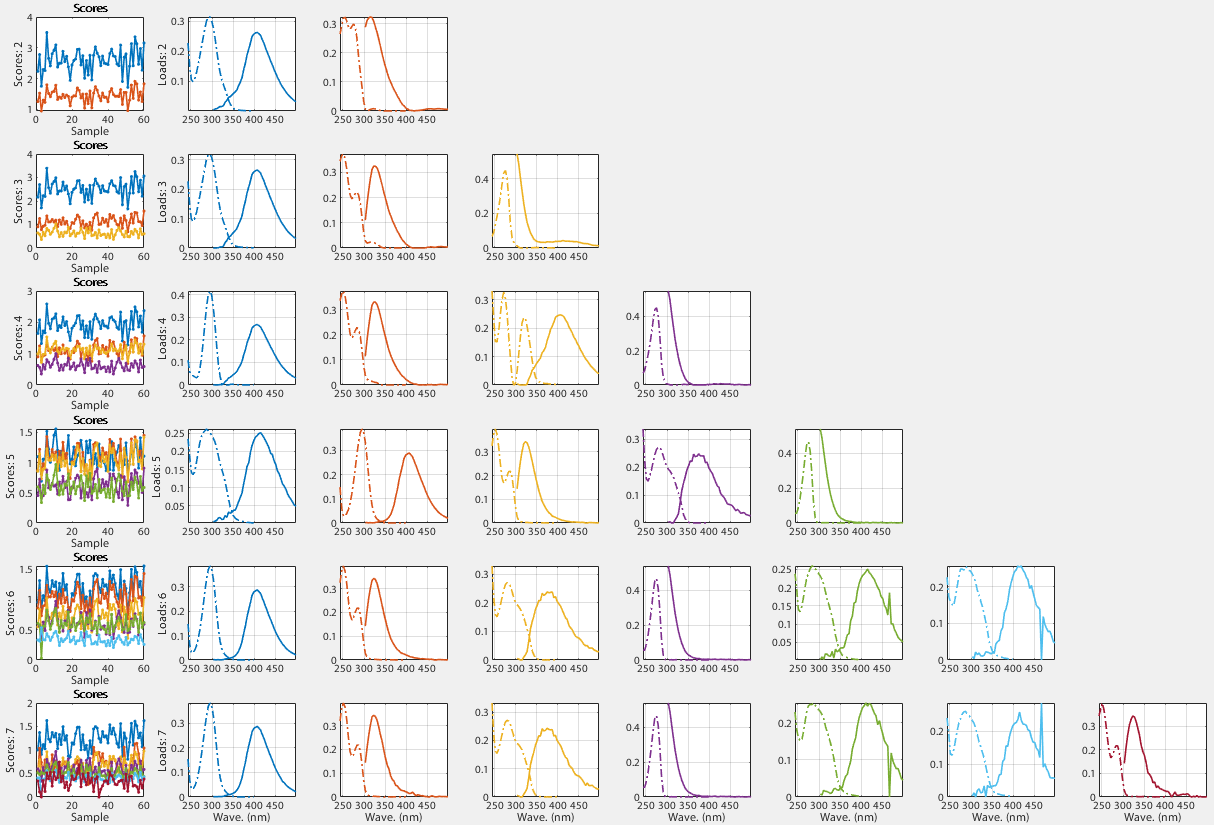What is shown here are the scores and loadings of PARAFAC models with three to seven components. You can obtain this plot again by calling `spectralloadings(models,2:7)`. Notice that in the six- and seven-component model, some of the components show abruptly decreasing or increasing loadings? That’s some bad emission scan that wasn’t removed before.

To diagnose this issue, we have a function called `loadingsandleverages`. Type `doc loadingsandleverages` to learn more. We want to take a look at the six-component model:

``````loadingsandleverages(models,6)
``````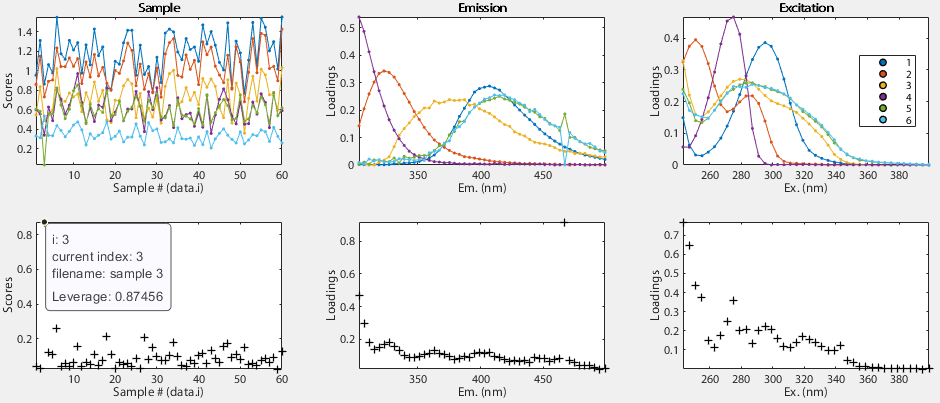It seems that sample 3 has a really high leverage. Use `eemreview` and `zap` to address this.

``````DS=zap(DS,3,467,[]);
``````

With that, we went one step back (outlier diagnosis) and therefore have to re-fit the two- to seven-component models. Don’t worry about overwriting the previous model. It is now useless.

``````models=randinitanal(DS,2:7,10,'nonnegativity');
``````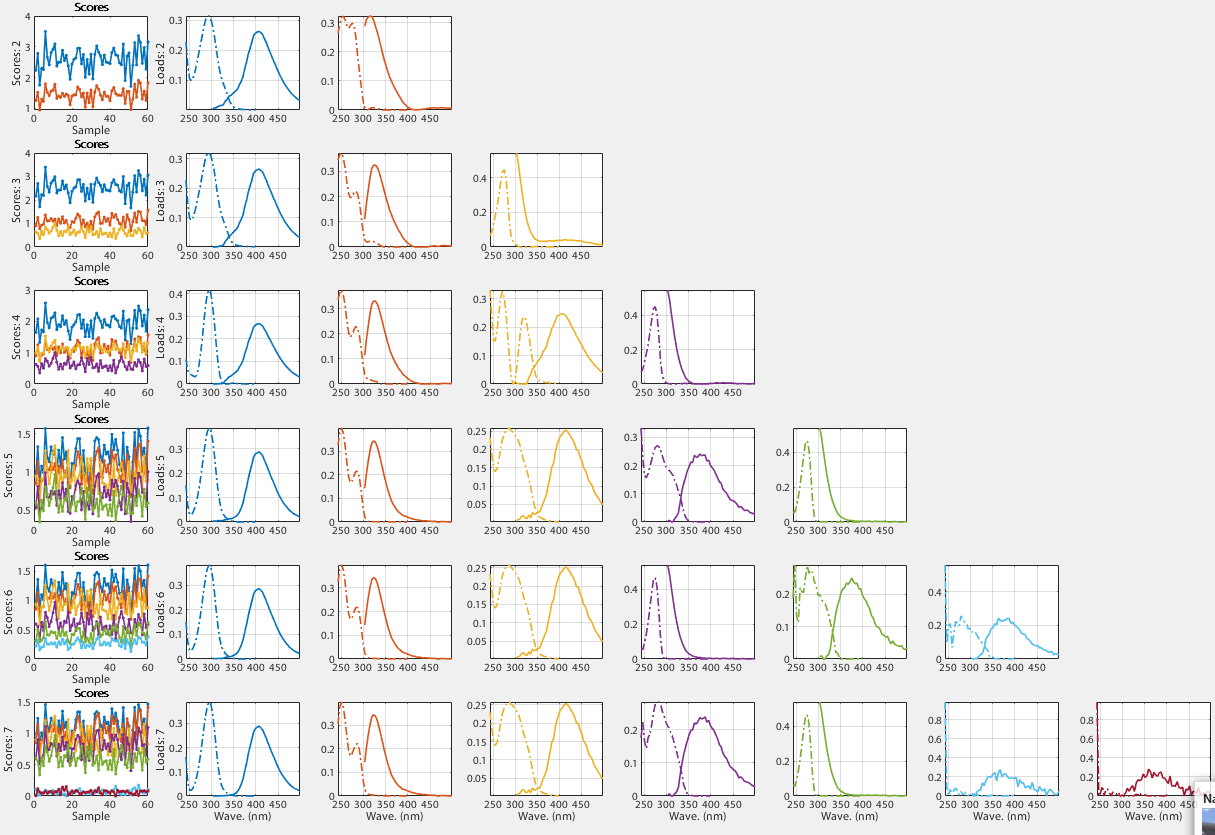Now, the loadings look better. Starting with the six-component model, we see non-smooth excitation loadings for some components. These components also have very low scores (take a look at the left-most plots). Is this another outlier causing trouble? Let’s take a look:

``````loadingsandleverages(models,6)
``````

This time, none of the sample have high leverages. Perhaps the strange excitation loadings were a sign of overfitting? Overfitting is when a PARAFAC model has too many components, which produces invalid models. We have some diagnostics to tell if this may be happening. A nice way of telling is to compare the core consistency of models with their explained variance. `coreandvar` helps us to visualize this information. Learn more about the core consistency here

``````coreandvar(models)
``````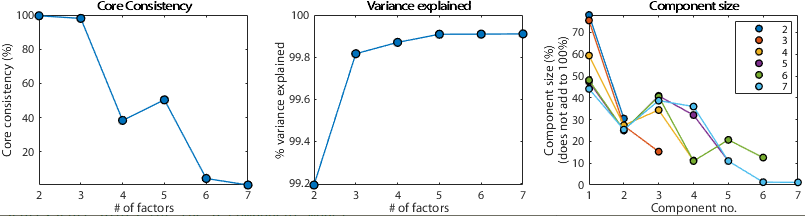We see that PARAFAC explains a lot more variance when we add components up until five components. The six-component model does not explain much more variance. At the same time, the core consistency drops from around 40-50% to 10%. This is a clear sign that five components are enough to describe the dataset. Remember that we did not like the 6th component of the six-component model and we could not figure out why it looked strange. This is even more evidence that five components are enough here.

Let’s take another close look at the five-component model. From `coreandvar` we know it describes 99.9% of the dataset. How do the residuals look? What does not model fail to explain? First, we look at the sum of squared errors. These are always positive (squared & summed).

``````specsse(models,5)
``````

This looks like very random residuals. Let’s take a closer look:

``````eemview({models,models},{'X','Model5','error_residuals'},[4 3],[],[],{'data','model','error'},[1 1 0.3],[],'colorbar')
``````

Very nice! These residuals are really random. If you model DOM fluorescence, these plots will likely not look as perfect. DOM fluorescence is more complicated. The core consistency tends to be over-cautious, and residuals look less random. Read more about the DOM-specific challenges here.

If you ran all these lines of code, you may have come across a warning dialogue that told you that the factors were highly correlated. This can be an issue in PARAFAC-modeling and in the worst case produces invalid models (read here to find out why). In drEEM, we can diagnose the model model scores with `compcorrplot`. Excellent correlations between components is a bad sign.

``````compcorrplot(models,5)
``````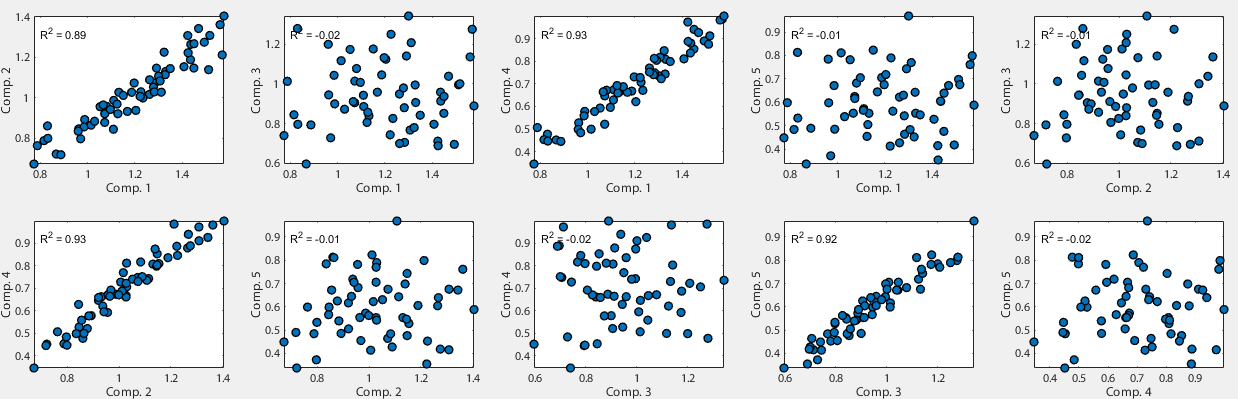We can see that components 1 and 2, 1 and 4, 2 and 4, and 3 and 5 are highly correlated. Luckily, we can try to reduce correlations by dividing EEMs by their sum of fluorescence. This forces PARAFAC to focus on qualitiative changes over quantitative changes in EEMs.

``````DSn=normeem(DS);
``````

Now, we have to run `randinitanal` again (the data has changed again). But let’s focus on 4-6 components to save time.

``````modelsn=randinitanal(DSn,4:6,10,'nonnegativity');
``````

Let’s look at the correlation plot again.

``````compcorrplot(modelsn,5)
``````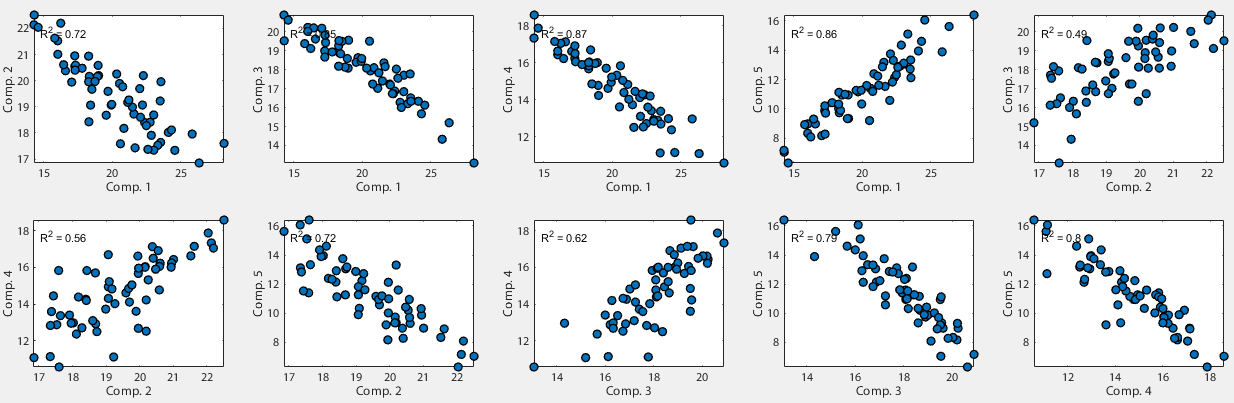That seems to have made things worse! Normalizing EEMs can sometimes help, but sometimes it also introduces correlations that did not exist before. So it is important to note that using `normeem` is an option, not a routine step.

## Validate the model

We have settled for a five component model and made sure that our data is in order by looking carefully for outliers. Now it’s time to validate this model. First, we should make sure that we have obtained not some random solution, but the best PARAFAC solution possible. This is called the “global minimum”. We do this by fitting a lot of models with five components and choosing the best one. If only 5 models are fit, there is a chance we work with a random solution that is -in fact- not the correct one.

Now is a good time to have Fika, as 100 five-component models will take some time. But it is well worth waiting and not cutting the number down to 20 models. Again, the more physical cores your computer’s CPU has, the faster this will go.

``````LSmodel=randinitanal(DS,5,100,'nonnegativity');
``````

Next, we attempt a split-half validation. This test investigates whether our PARAFAC solution found in `LSmodel` is also found when only half (or less of) the data is supplied. It is a good idea to read the documentation of splitds (`doc splitds`) to learn about all your options. Here, we split the data randomly into six splits and recombine two to form three final splits with 20 samples each.

``````DSsplit=splitds(DS,[],6,'random',{[1 2],[3 4],[5 6]})
``````

Afterwards, we fit 50 PARAFAC models with 5 components (to find the best solution) and do this for each split. That is 3*50 = 150 models total. Perhaps tea-time? The good news is that each model will take less time with less samples.

``````splitmodels=splitanalysis(DSsplit,5,50,'nonnegativity');
``````

No graphical output is created here. The actual validation happens with `splitvalidation`.

``````val_results=splitvalidation(splitmodels,5,[],[],LSmodel);
``````

If you are lucky you read

``````>> val_results=splitvalidation(splitmodels,5,[],[],LSmodel);
Overall Result= Validated for all comparisons

The final rows of ExCC, EmCC and R (i.e. R{end}, ExCC{end}, EmCC{end}
compare each split included in the validation against the overall model.
Note that the order of results (left to right) for this comparison
corresponds to the split order shown in Val_Splits:
1     2     3
``````

And you will see this output: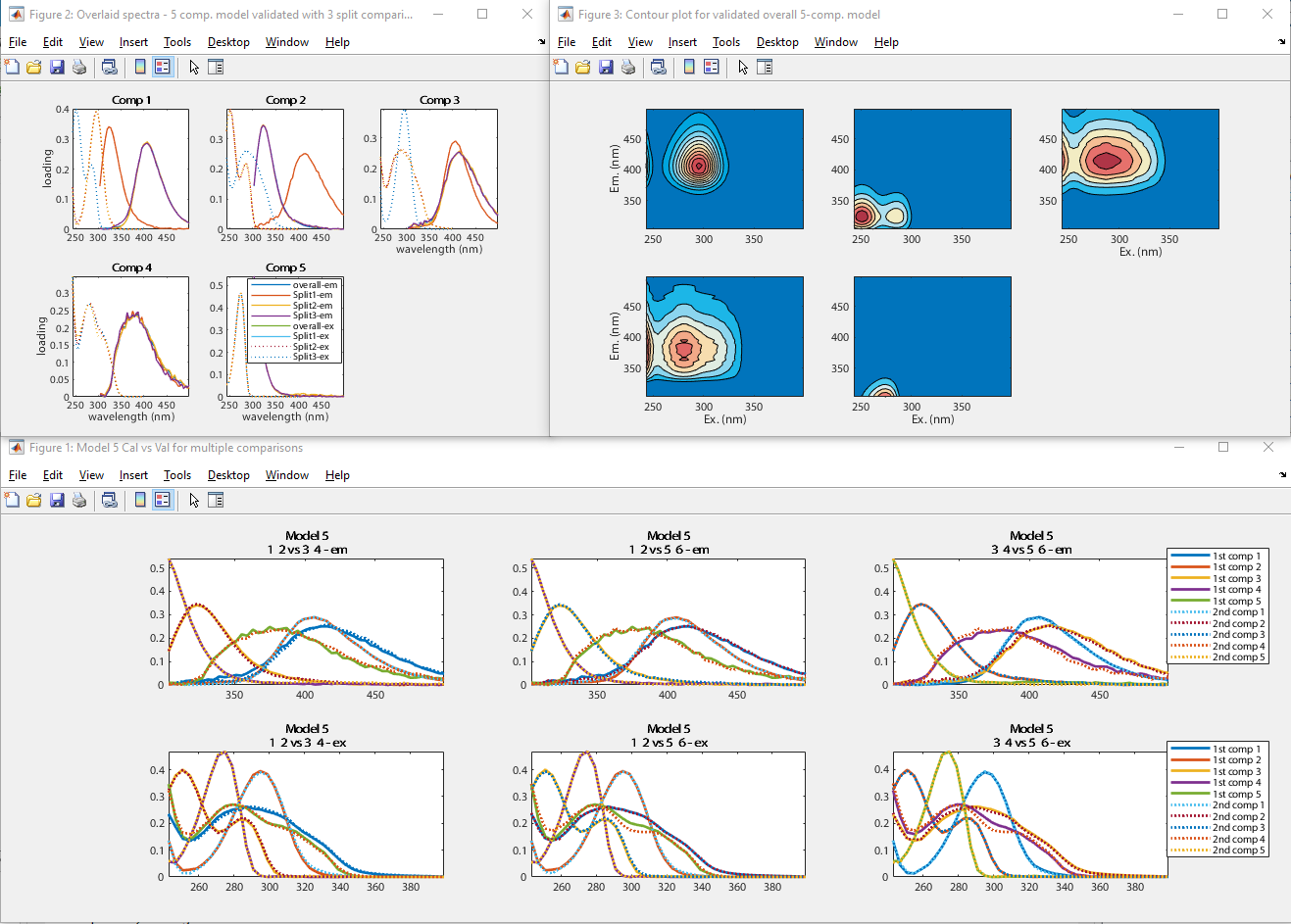This is to let us know of the details. In all comparisons, PARAFAC fit models that were so similar that they are considered equal. This is the sign of a successful split-half validation.

## Export the model

Now that we found a valid PARAFAC model, we can export the results. In drEEM, you have two options.

### Export a model to excel

If you wish to share the model with your collaborators or are more familiar with working in Microsoft Excel, you may want to export a model to an Excel file. drEEM lets you do this with the function `modelout`(Windows) or `modeloutmac` (macOS).

``````[FMax,B,C] = modelout(val_results,5,'pure_5_validated.xlsx');
``````

This Excel-workbook contains a report informing you about the dataset and a Fmax and loadings of all components.

In 2014, a open database was created for sharing and comparing your NOM / DOM PARAFAC results. This database is called OpenFluor, and is located at http://openfluor.org. There, you can privately upload your models and compare them to previously published datasets. This aids the interpretation of your own model as it helps you to understand what other people have found in connection to fluorescent components. On the website, you upload a text-file, so we first have to create it. The function `openfluor` does this for us.

``````openfluor(val_results,5,'pure_5_validated_of.txt')
``````

This function does not export scores, but only the loadings of your components. Until you publish your data, no one will be able to see your data. For a tutorial of the new website, click here. If you would like your data to stay on your computer, you can use OpenFluor for OpenChrom (video).

Once you have performed a model comparison on OpenFluor, you have the option to export matches: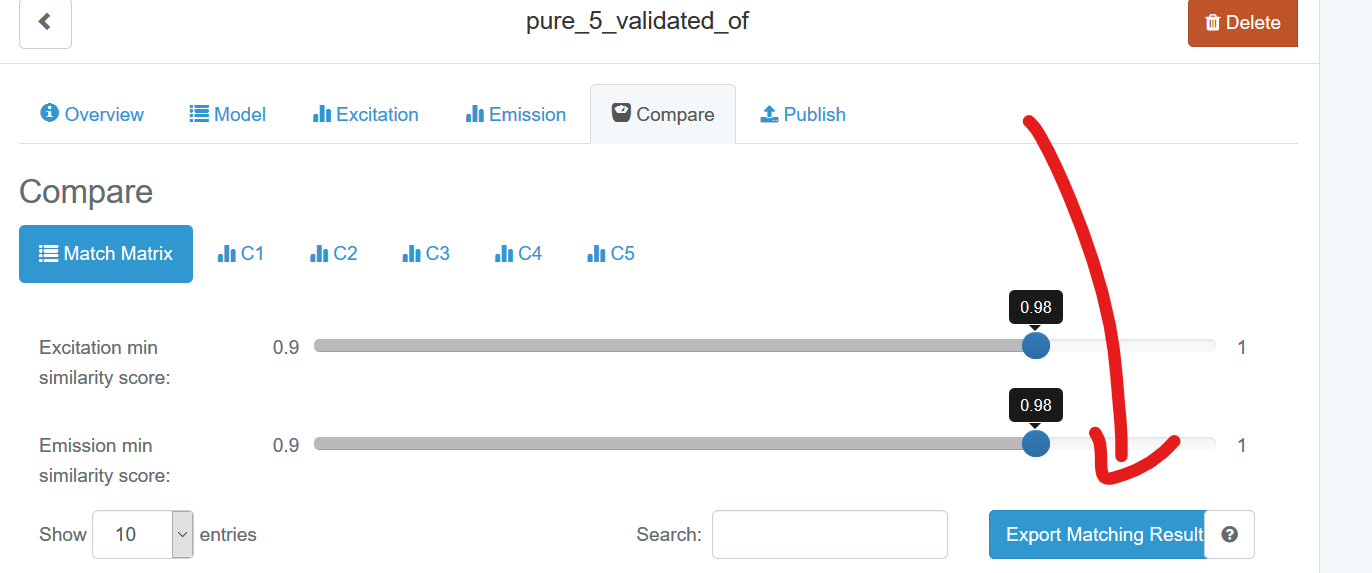Since drEEM v0.6.0, you can import the match results back into MATLAB to get nice overview plots and to dive deeper into component matches. The function that does this is called `openfluormatches`. I downloaded matches after restricting the criteria to TCC >0.98 for both excitation and emission loadings. I then run:

``````% To make this line work, you will need to carry out your own upload and comparison and modify the path in the line below.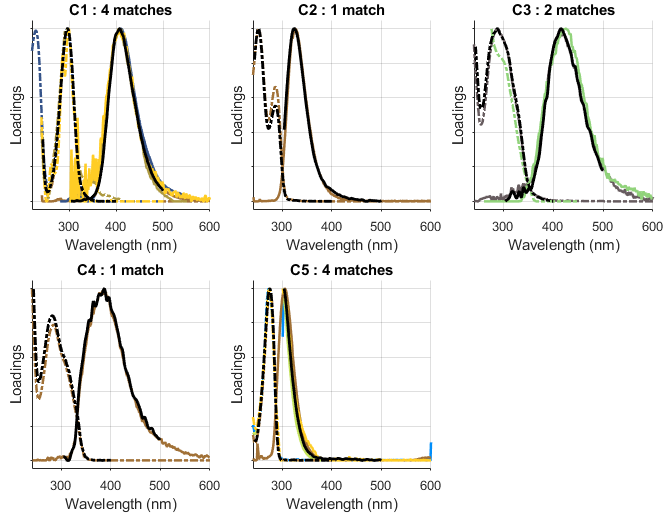Note that you can zoom in, click on the spectra & when the users uploaded their models incl. the article DOI, the DOI will be copied directly to the clipboard. In your browser, you simply press `Ctrl + V` (Windows) or `command + V` (macOS) and you will be taken to the article. When I do this for C4, I see a match with “OrganicCompouds1 (C7)”.